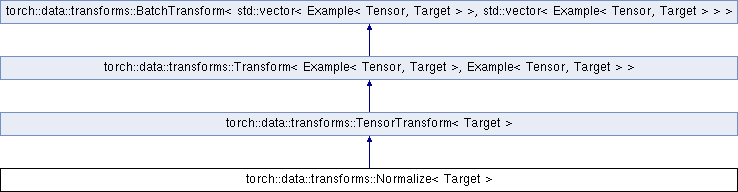Caffe2 - C++ API A deep learning, cross platform ML framework
torch::data::transforms::Normalize< Target > Struct Template Reference

Normalizes input tensors by subtracting the supplied mean and dividing by the given standard deviation. More...

`#include <tensor.h>`

Inheritance diagram for torch::data::transforms::Normalize< Target >:## Public Member Functions

Normalize (ArrayRef< double > mean, ArrayRef< double > stddev)
Constructs a `Normalize` transform. More...

torch::Tensor operator() (Tensor input)
Transforms a single input tensor to an output tensor.Public Member Functions inherited from torch::data::transforms::TensorTransform< Target >
OutputType apply (InputType input) override
Implementation of `Transform::apply` that calls `operator()`.Public Member Functions inherited from torch::data::transforms::Transform< Example< Tensor, Target >, Example< Tensor, Target > >
std::vector< Example< Tensor, Target > > apply_batch (std::vector< Example< Tensor, Target > > input_batch) override
Applies the `transformation` over the entire `input_batch`.

## Data Fields

torch::Tensor mean

torch::Tensor stddev

## Additional Inherited MembersPublic Types inherited from torch::data::transforms::TensorTransform< Target >
using E = Example< Tensor, Target >Public Types inherited from torch::data::transforms::Transform< Example< Tensor, Target >, Example< Tensor, Target > >
using InputType = Example< Tensor, Target >

using OutputType = Example< Tensor, Target >Public Types inherited from torch::data::transforms::BatchTransform< std::vector< Example< Tensor, Target > >, std::vector< Example< Tensor, Target > > >
using InputBatchType = std::vector< Example< Tensor, Target > >

using OutputBatchType = std::vector< Example< Tensor, Target > >

## Detailed Description

### template<typename Target = Tensor> struct torch::data::transforms::Normalize< Target >

Normalizes input tensors by subtracting the supplied mean and dividing by the given standard deviation.

Definition at line 57 of file tensor.h.

## Constructor & Destructor Documentation

template<typename Target = Tensor>
 torch::data::transforms::Normalize< Target >::Normalize ( ArrayRef< double > mean, ArrayRef< double > stddev )
inline

Constructs a `Normalize` transform.

The mean and standard deviation can be anything that is broadcastable over the input tensors (like single scalars).

Definition at line 61 of file tensor.h.

The documentation for this struct was generated from the following file:
• torch/csrc/api/include/torch/data/transforms/tensor.h